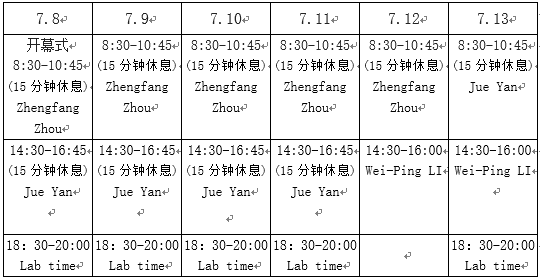## 关于2019年浙江大学求是数学暑期学校的通知Introduction to Algebraic Geometry

Wei-Ping LI（香港科技大学）

This is an introductory course of algebraic geometry. We start with geometric aspect of algebraic geometry. Here we introduce the concept of complex manifolds, divisors, line bundles, the canonical divisor, adjunction formula, sheaves and cohomology of sheaves. Some concrete examples such as Riemann surfaces, called algebraic curves, and algebraic surfaces will be discussed. Riemann-Hurwitz formula and Riemann-Roch formula will be studied if time permits. This is in the spirit of Griffiths and Harris’ book "Principles of Algebraic Geometry”.

Numerical Methods for Ordinary Differential Equations/Systems

Jue YanIowa State University

In this course we will carry out a comprehensive study on finite difference numerical methods solving ODEs. We will start with first order Euler method and introduce the concept of local truncation error and consistency of a method. We then review function approximation with Lagrange interpolation polynomial and its error term. High order multi-step method will be studied with the assistance of Lagrange polynomial approximation. High order multi-stage Runge-Kutta method will be derived. Toward the end we will introduce zero-stability concept and finalize the discussion with absolute-stability for long time run for dynamic systems.

First order Euler method Matlab sample code will be given before the class. Two home works (high order multi-step method and high order Runge-Kutta method) are expected and will be carried out in two-body groups format. Daily Lab time attendance is expected and graduate student assistance will be available on MatLab code implementation.

Lectures on Fixed Point Theory and Its Applications

Zhengfang Zhou (Michigan State University)

Many mathematical problems can be formulated as solutions of an equation F(x) = 0 , or equivalently x = G(x) = x - F(x), namely fixed points for a function. The lectures will focus on the fixed point theory, starting from the simple contraction principle, famous Brouwer fixed point theorem, then going to topological degree theory for fnite dimensions and general Banach spaces. We will also study the index theory for symmetrical functions, and establish some existence theory for multiple solutions. Finally we will use the theory to study the existence and multiple or infinitely many solutions of some nonlinear partial differential equations. The depth and breadth of the application will depend on the background of students. Most of the lectures are in English, and students are encouraged to ask questions and actively participate the discussion during the classes.

《求是数学导论系列讲座》彭赛列闭合定理
李时璋 助理教授（密西根大学安娜堡分校）